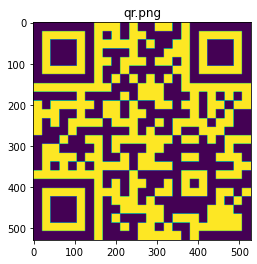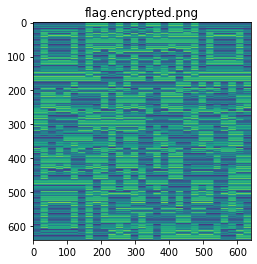## Challenge

You are given a scrambled QR code that looks like it has been encrypted with ECB (Electronic Code Book Mode).## Solution

The encryption is done in chunks of height 16, even though the QR-code itself has squares of a size of roughyl 22x22 for the 2nd and 21x21 for the 1st.

generate_lookup: As we have a known plaintext, we can extract encrypted chunks and build a lookup table. As the chunks are exactly the same along the x-axis, we can only sample the center of the chunk for this lookup table, so at pos 11, 33, 55, 77 for width 22.

apply_lookup: The lookup-table generated from `qr.png` to `qr.encrypted.png` can be used to reverse most chunks of height 16 in `flag.encrypted.png`. In total, 4 chunks can’t be resolved (found by logging accesses to lookup in apply_lookup) -> we fill them with grey, and hope that we get the required info from the 16x16 chunk above.

fix_qr: This fix_qr simply checks, if a 22x22 qr-chunk has at least one pixel set to 255, then it sets the whole chunk to 255. Otherwise to 0 - effectively interpolating ignoring the grey chunk.

To extract the result with pyzbar, we have to rescale the image, but the rescale from cv2 interpolates. The kronecker-product combined with ones can simply repeat pixels to make an exact upscaling.

``````import matplotlib.pyplot as plt
import cv2
import numpy as np
from collections import defaultdict

plt.title(fname)
plt.imshow(img)
plt.show()
return img

i1_clr, i1_enc, i2_enc = map(read_and_plot, ["qr.png", "qr.encrypted.png", "flag.encrypted.png"])
````````````# CONSTANTS
SIZE1 = 25 # number of QR-chunks in 1st image
SIZE2 = 29 # number of QR-chunks in the 2nd encrypted image

CHEIGHT = 16  # encryption chunk height
CHEIGHT2 = 22  # QR chunk height

FSIZE = CHEIGHT2 * SIZE2  # corrected size, so all chunks are equally big
``````
``````def build_lookup(img_clr, img_enc, SIZE):
""" build lookup dict from enc to clear strips (16 -> 16) and defaults to 128 """
HEIGHT = img_clr.shape  # image height/width in px
CWIDTH = int(HEIGHT/SIZE)  # chunk width

lookup = defaultdict(lambda: 128)
for y in range(0, HEIGHT, CHEIGHT):
for xpos in range(SIZE):
x = int(xpos*(HEIGHT/SIZE) + CWIDTH/2)  # center line of a chunk
block_enc = img_enc[y:y+CHEIGHT, x]
block_clr = img_clr[y:y+CHEIGHT, x]
lookup[tuple(block_enc)] = block_clr
return lookup

def apply_lookup(img_enc, lookup, SIZE):
""" apply lookup table and replace encrypted with cleartex chunks and unknown with 128 """
HEIGHT = img_enc.shape  # image height/width in px
CWIDTH = int(HEIGHT/SIZE)  # chunk width

# build image of 640 x 29 with looked up chunks
img = np.zeros((HEIGHT, SIZE))
for y in range(0, HEIGHT, CHEIGHT):
for x in range(SIZE):
xstart = int((x+0.5)*CWIDTH)
block_enc = img_enc[y:y+CHEIGHT, xstart]
img[y:y+16, x] = lookup[tuple(block_enc)]
return img

def fix_qr(img, SIZE):
""" fix unknown chunks by checking if the chunk contains the max value """
img = cv2.resize(img, (SIZE, FSIZE))  # fix on the basis of qr-code chunks
for y in range(0, FSIZE, CHEIGHT2):
for x in range(0, SIZE):
chunk = img[y:y+CHEIGHT2, x]
img[y:y+CHEIGHT2, x] = 255 * (chunk.max() == 255)
return cv2.resize(img, (SIZE, SIZE))  # resize to 29 x 29

def upscale(img, x=1, y=1): # repeat pixels x-times in x-dim and y-times in y-dim with kronecker-product
return np.kron(img, np.ones((x, y)))

lookup = build_lookup(i1_clr, i1_enc, SIZE1)

img = apply_lookup(i2_enc, lookup, SIZE2)
big_img = upscale(img, 1, CHEIGHT2)
plt.title("Intermediate results with unknown half-chunks")
plt.imshow(big_img)
plt.show()

img = fix_qr(img, SIZE2)
plt.title("Final result")
plt.imshow(img)
plt.show()
big_img = upscale(img, CHEIGHT2, CHEIGHT2)
cv2.imwrite("flag.png", big_img);
````````````# pyzbar can't handle images where the QR-code-chunks size is exactly 1, so we needed to upscale
from pyzbar.pyzbar import decode
decoded = decode(big_img)
print(decoded.data.decode(), decoded, sep="\n\n")
``````
``````VolgaCTF{S0m3tim35_3C8_c4n_b3_t00_pr3dict4b13}

[Decoded(data=b'VolgaCTF{S0m3tim35_3C8_c4n_b3_t00_pr3dict4b13}', type='QRCODE', rect=Rect(left=-1, top=-1, width=640, height=640), polygon=[Point(x=-1, y=-1), Point(x=-1, y=639), Point(x=638, y=638), Point(x=639, y=-1)])]
``````